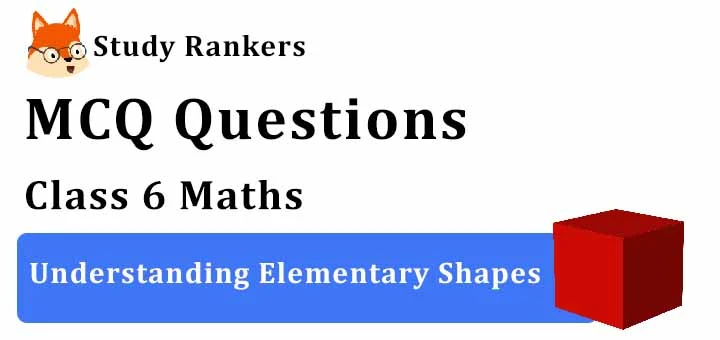## MCQ Questions for Class 6 Maths: Ch 5 Understanding Elementary Shapes1. How many centimetres make 3m?
(a) 100
(b) 30
(c) 300
(d) 3000
► (c) 300

2. What fraction of a clockwise revolution does the hour hand of a clock turn through, when it goes from 1 to 10?
(a) 3/4
(b) 1/4
(c) More than 3/4
(d) none of these
► (a) 3/4

3. Where will the hand of a clock stop if it starts at 2 and makes 1/2 of a revolution, clockwise?
(a) 5
(b) 8
(c) 11
(d) None of these
► (b) 8

4. When an arm of an angle is extended then how does its measure change?
(a) Doubled
(b) Tripled
(c) Remains the same
(d) Halved
► (c) Remains the same

5. Where will the hand of a clock stop if it starts at 12 and makes 3/4 of a revolution, clockwise?
(a) 6
(b) 9
(c) 3
(d) None of these
► (b) 9

6. An angle whose measure is equal to half of a revolution is
(a) right angle
(b) acute angle
(c) straight angle
(d) obtuse angle
► (c) straight angle

7. What fraction of a clockwise revolution does the hour hand of a clock turn through, when it goes from 5 to 11?
(a) 1/2
(b) More than 1/4
(c) 3/4
(d) none of these
► (a) 1/2

8. When the sum of the measures of two angles is that of a right angle, then each one of them is ______.
(a) obtuse angle
(b) acute angle
(c) straight angle
(d) right angle
► (b) acute angle

9. At 5:20 what type of angle is formed between the two hands of a clock?
(a) An obtuse angle
(b) A right angle
(c) An acute angle
(d) A reflex angle
► (c) An acute angle

10. An angle whose measure is equal to one-fourth of a revolution is
(a) right angle
(b) straight angle
(c) obtuse angle
(d) acute angle
► (a) right angle

11. An angle whose measure is equal to a full revolution is
(a) complete angle
(b) right angle
(c) obtuse angle
(d) straight angle
► (a) complete angle

12. 179° is an example of which of these angles?
(a) An obtuse angle
(b) An acute angle
(c) A right angle
(d) A straight angle
► (a) An obtuse angle

13. How is the measure of an angle expressed?
(a) Compasses
(b) Protractor
(c) Degrees
(d) Centimetres
► (c) Degrees

14. An angle whose measure is greater than that of a right angle is ______.
(a) right angle
(b) straight angle
(c) acute angle
(d) obtuse angle
► (d) obtuse angle

15. What fraction of a clockwise revolution does the hour hand of a clock turn through, when it goes from 7 to 10?
(a) 1/2
(b) 1/4
(c) 1/3
(d) None of these
► (b) 1/4

16. When the sum of the measures of two angles is that of a straight angle and if one of them is acute then the other should be _______.
(a) obtuse
(b) straight
(c) right
(d) acute
► (a) obtuse

17. l and m are two lines perpendicular to each other. What is the measure of the angle between them?
(a) 10°
(b) 50°
(c) 40°
(d) 90°
► (d) 90°

18. What fraction of a clockwise revolution does the hour hand of a clock turn through, when it goes from 3 to 9?
(a) 1/3
(b) 1
(c) 1/4
(d) 1/2
► (d) 1/2

19. What is an angle which measures more than 0∘ and less than 90∘ called?
(a) Obtuse angle
(b) Acute angle
(c) Right angle
(d) Straight angle
► (b) Acute angle

20. What fraction of a clockwise revolution does the hour hand of a clock turn through, when it goes from 3 to 6?
(a) 1/4
(b) 1
(c) 1/2
(d) None of these
► (a) 1/4

21. Where will the hand of a clock stop if it starts at 3 and makes 3/4 of a revolution, clockwise?
(a) 6
(b) 12
(c) 9
(d) None of these
► (b) 12

22. Where will the hand of a clock stop if it starts at 6 and makes 3/4 of a revolution, clockwise?
(a) 3
(b) 12
(c) 9
(d) 6
► (a) 3